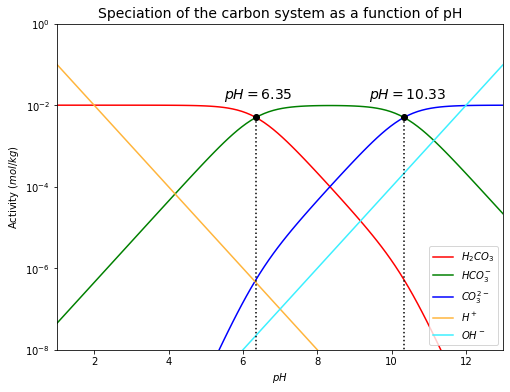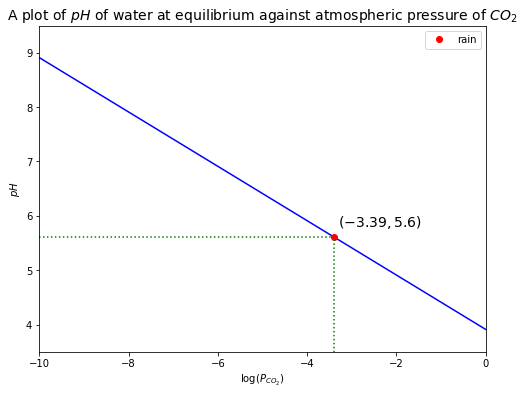# CO2-H2O System#

Low-Temperature Geochemistry

In this session, we will derive the governing equations of the $$CO_2$$-$$H_2O$$ system under closed and open system conditions.

# import relevant modules

%matplotlib inline
import numpy as np
import matplotlib.pyplot as plt
import pandas as pd
from IPython.display import display

%%javascript
MathJax.Hub.Config({
TeX: { equationNumbers: { autoNumber: "AMS" } }
});
MathJax.Hub.Queue(
["resetEquationNumbers", MathJax.InputJax.TeX],
["PreProcess", MathJax.Hub],
["Reprocess", MathJax.Hub]
);


Consider the dissociation of a weak poly-protic acid ($$H_2CO_3$$) in water at $$25^\circ C$$ and an atmospheric pressure of $$1$$ bar.

(1) $$H_2CO_3$$ dissociates to form hydrogen ions ($$H^+$$) and bicarbonate ions ($$HCO_{3}^{-}$$)

$H_2CO_3 = HCO_{3}^{-} + H^+$
(292)#$K_1 = \frac{a_{H^+} \cdot a_{HCO_{3}^{-}}}{a_{H_2CO_3}} = 10^{-6.35}$

(2) $$HCO_{3}^{-}$$ dissociates to form hydrogen ions and carbonate ions ($$CO_{3}^{2-}$$)

$HCO_{3}^{-} = CO_{3}^{2-} + H^+$
(308)#$K_2 = \frac{a_{H^+} \cdot a_{CO_{3}^{2-}}}{a_{HCO_{3}^{-}}} = 10^{-10.33}$

(3) Dissociation of water

$H_2O = H^+ + OH^-$
(309)#$K_W = \frac{a_{H^+} \cdot a_{OH^-}}{a_{H_2O}} = 10^{-14}$

(4) Charge balance (all solutions are electrically neutral). Note that we need to balance concentrations ($$m$$), not activities ($$a$$), as concentrations represent actual quantities of ions.

(310)#$m_{H^+} = m_{HCO_{3}^{-}} + 2m_{CO_{3}^{2-}} + m_{OH^-}$

Now we have $$5$$ unknowns ($$m_{H^+}, m_{H_2CO_3}, m_{HCO_{3}^{-}}, m_{CO_{3}^{2-}}, m_{OH^-}$$) but only $$4$$ equations. We need the fifth equation in order to solve the simultaneous equations. Fortunately, we can get it from the boundary conditions, i.e. what we know about the system.

For a closed system, the boundary condition is that the total amount of $$CO_2$$ in the system is fixed. As $$H_2CO_3$$, $$HCO_{3}^{-}$$, and $$CO_{3}^{2-}$$ form when $$CO_2$$ dissolves in water, so

(296)#$m_{total} = m_{H_2CO_3} + m_{HCO_{3}^{-}} + m_{CO_{3}^{2-}}$

Recalling the relationship between concentration and activity:

$m_i = \frac{a_i}{y_i}$

We will assume that $$y_{H_2CO_3} = y_{HCO_{3}^{-}} = y_{CO_{3}^{2-}} = y_{OH^-} = 1$$, so $$m_i = a_i$$.

And recall that

$pH = -\log(m_{H^+}) \quad \longrightarrow \quad m_{H^+}=10^{-pH}$

From (292), $$m_{H^+}=10^{-pH}$$, rearranging it and using the assumption, we get:

(297)#$m_{HCO_{3}^{-}} = \frac{K_1 \cdot m_{H_2CO_3}}{10^{-pH}}$

From $$m_{H^+}=10^{-pH}$$, (308) and (297), using the assumption, we get:

(298)#$m_{CO_{3}^{2-}} = \frac{K_2 \cdot K_1 \cdot m_{H_2CO_3}}{10^{-2 \cdot pH}}$

Substituting (297) and (298) into (296), we get:

$m_{total} = m_{H_2CO_3} + \frac{K_1 \cdot m_{H_2CO_3}}{10^{-pH}} + \frac{K_2 \cdot K_1 \cdot m_{H_2CO_3}}{10^{-2 \cdot pH}}$
$m_{total} = m_{H_2CO_3} \left(\frac{K_1}{10^{-pH}} + \frac{K_2 \cdot K_1}{10^{-2 \cdot pH}}\right)$
$m_{H_2CO_3} = \frac{m_{total}}{\left(\frac{K_1}{10^{-pH}} + \frac{K_2 \cdot K_1}{10^{-2 \cdot pH}}\right)}$

We will define a new parameter $$F_H$$ as

$F_H = \frac{K_1}{10^{-pH}} + \frac{K_2 \cdot K_1}{10^{-2 \cdot pH}}$

So,

(299)#$m_{H_2CO_3} = \frac{m_{total}}{F_H}$

Substituting (299) into (297) and (298), we get:

(300)#$m_{HCO_{3}^{-}} = \frac{m_{total} \cdot K_1}{F_H \cdot 10^{-pH}}$
(301)#$m_{CO_{3}^{2-}} = \frac{m_{total} \cdot K_2 \cdot K_1}{F_H \cdot 10^{-2 \cdot pH}}$

The derivations of (299), (300) and (301) lead to the speciation of the carbon system as a function of $$pH$$, as shown below.

# function for calculating F_H, m_H2CO3, m_HCO3-, m_CO3--, m_H+, m_OH-
def FH(pH):
K1 = 10**-6.35
K2 = 10**-10.33
return 1+(K1/(10**-pH))+(K2*K1/(10**(-2*pH)))

def m_H2CO3(m_total, pH):
return m_total/FH(pH)

def m_HCO3(m_total, pH):
K1 = 10**-6.35
return m_total*K1/(FH(pH)*(10**-pH))

def m_CO3(m_total, pH):
K1 = 10**-6.35
K2 = 10**-10.33
return m_total*K2*K1/(FH(pH)*(10**(-2*pH)))

def m_H(pH):
return 10**-pH

def m_OH(pH):
return 10**-(14-pH)

# plot
plt.figure(figsize=(8,6))
m_total = 0.01  # set m_total value
pH = np.linspace(1, 13, 100)
plt.plot(pH, m_H2CO3(m_total, pH), 'r', label='$H_2CO_3$')  # H2CO3
plt.plot(pH, m_HCO3(m_total, pH), 'g', label='$HCO_{3}^{-}$')  # HCO3-
plt.plot(pH, m_CO3(m_total, pH), 'b', label='$CO_{3}^{2-}$')  # CO3--
plt.plot(pH, m_H(pH), '#FFB53C', label='$H^+$')  # H+
plt.plot(pH, m_OH(pH), '#3CF0FF', label='$OH^-$')  # OH-
plt.plot(6.35, m_H2CO3(m_total, 6.35), 'ko')  # the point where m_H2CO3=m_HCO3-
plt.plot([6.35, 6.35], [0, m_H2CO3(m_total, 6.35)], 'k:')
plt.text(5.5, 0.015, '$pH=6.35$', fontsize=14)
plt.plot(10.33, m_HCO3(m_total, 10.33), 'ko')  # the point where m_HCO3-=m_CO3--
plt.plot([10.33, 10.33], [0, m_HCO3(m_total, 10.33)], 'k:')
plt.text(9.4, 0.015, '$pH=10.33$', fontsize=14)
plt.xlabel('$pH$')
plt.ylabel('Activity $(mol/kg)$')
plt.xlim([1, 13])
plt.ylim([10**-8, 1])
plt.yscale("log")
plt.title('Speciation of the carbon system as a function of pH', fontsize=14)
plt.legend(loc='best', fontsize=10)

<matplotlib.legend.Legend at 0x1fd60a9e460>From the plot above, it can be seen that there are two points where the concentrations (activities) of two carbon-bearing species are equal.

1. $$m_{H_2CO_3} = m_{HCO_{3}^{-}}$$

$\frac{(a)}{(b)} = \frac{m_{H_2CO_3}}{m_{HCO_{3}^{-}}} = \frac{\left(\frac{m_{total}}{F_H}\right)}{\left(\frac{m_{total} \cdot K_1}{F_H \cdot 10^{-pH}}\right)} = \frac{10^{-pH}}{K_1}$

$$\quad$$If $$m_{H_2CO_3} = m_{HCO_{3}^{-}}$$, so

$\frac{10^{-pH}}{K_1} = 1$
$\therefore pH = pK_1 = 6.35$
1. $$m_{HCO_{3}^{-}} = m_{CO_{3}^{2-}}$$

$\frac{(b)}{(c)} = \frac{m_{HCO_{3}^{-}}}{m_{CO_{3}^{2-}}} = \frac{\left(\frac{m_{total} \cdot K_1}{F_H \cdot 10^{-pH}}\right)}{\left(\frac{m_{total} \cdot K_2 \cdot K_1}{F_H \cdot 10^{-2 \cdot pH}}\right)} = \frac{10^{-pH}}{K_2}$

$$\quad$$If $$m_{HCO_{3}^{-}} = m_{CO_{3}^{2-}}$$, so

$\frac{10^{-pH}}{K_2} = 1$
$\therefore pH = pK_2 = 10.33$

Next, we will consider an open system at $$25^\circ C$$ and an atmospheric pressure of $$1$$ bar with a constant atmospheric partial pressure of $$CO_2$$ ($$pCO_2$$)

Some $$CO_2$$ in the air will dissolve in water. The amount of dissolved $$CO_2$$ follows Henry’s law.

$K_H \cdot pCO_2 = (CO_2)_{aq}$
(302)#$K_H = \frac{a_{(CO_2)_{aq}}}{P_{CO_2}}$

Some dissolved $$CO_2$$ will react with water to form carbonic acid:

$(CO_2)_{aq} + H_2O = H_2CO_3$
(303)#$K_0 = \frac{a_{H_2CO_3}}{a_{(CO_2)_{aq}} \cdot a_{H_2O}} = \frac{a_{H_2CO_3}}{a_{(CO_2)_{aq}}}$

Rearranging (302) and (303), we get:

(304)#$a_{(CO_2)_{aq}} = K_H \cdot P_{CO_2}$
(305)#$a_{H_2CO_3} = K_0 \cdot a_{(CO_2)_{aq}}$

Substituting (304) into (305), we get:

(306)#$a_{H_2CO_3} = K_0 \cdot K_H \cdot P_{CO_2}$

Combine both of the carbon-bearing species in water as $$H_2CO_3^*$$

$a_{H_2CO_3^* } = a_{(CO_2)_{aq}} + a_{H_2CO_3}$
$= K_H \cdot P_{CO_2} + K_0 \cdot K_H \cdot P_{CO_2}$
$= K_H \cdot (1+K_0) \cdot P_{CO_2}$

In reality, $$K_H >> K_0$$, so $$a_{(CO_2)_{aq}} >> a_{H_2CO_3}$$, and

$a_{H_2CO_3^*} \approx K_H \cdot P_{CO_2}$

Recall (292), (308), (309), (310), (302), but rewrite (292) and (302):

$H_2CO_3^* = HCO_{3}^{-} + H^+$
(307)#$K_1 = \frac{a_{H^+} \cdot a_{HCO_{3}^{-}}}{a_{H_2CO_3^{* }}} = 10^{-6.35}$
(308)#$K_2 = \frac{a_{H^+} \cdot a_{CO_{3}^{2-}}}{a_{HCO_{3}^{-}}} = 10^{-10.33}$
(309)#$K_W = \frac{a_{H^+} \cdot a_{OH^-}}{a_{H_2O}} = 10^{-14}$
(310)#$m_{H^+} = m_{HCO_{3}^{-}} + 2m_{CO_{3}^{2-}} + m_{OH^-}$
$K_H \cdot pCO_2 = H_2CO_3^{* }$
(311)#$K_H = \frac{a_{H_2CO_3^{* }}}{P_{CO_2}} \quad \longrightarrow \quad a_{H_2CO_3^{* }} = K_H \cdot P_{CO_2}$

Substituting (311) into (307), and rearranging, we get:

(312)#$a_{HCO_{3}^{-}} = \frac{K_1 \cdot K_H \cdot P_{CO_2}}{a_{H^+}}$

Substituting (299) into (308), and rearranging, we get:

(313)#$a_{CO_{3}^{2-}} = \frac{K_2 \cdot K_1 \cdot K_H \cdot P_{CO_2}}{a_{H^+}^2}$

Rearranging (309), we get:

(314)#$a_{OH^-} = \frac{K_W}{a_{H^+}}$

Applying $$m_i = \frac{a_i}{y_i}$$ to (310), we get:

$\frac{a_{H^+}}{y_{H^+}} = \frac{a_{HCO_{3}^{-}}}{y_{HCO_{3}^{-}}} + 2\cdot\frac{a_{CO_{3}^{2-}}}{y_{CO_{3}^{2-}}} + \frac{a_{OH^-}}{y_{OH^-}}$

Substituting (312), (313) and (314), we get:

$\frac{1}{y_{H^+}} \left(a_{H^+}\right) = \frac{1}{y_{HCO_{3}^{-}}} \left(\frac{K_1 \cdot K_H \cdot P_{CO_2}}{a_{H^+}}\right) + \cdot\frac{2}{y_{CO_{3}^{2-}}} \left(\frac{K_2 \cdot K_1 \cdot K_H \cdot P_{CO_2}}{a_{H^+}^2}\right) + \frac{1}{y_{OH^-}} \left(\frac{K_W}{a_{H^+}}\right)$

$$y_{H^+}, y_{HCO_{3}^{-}}, y_{CO_{3}^{2-}}$$, and $$y_{OH^-}$$ can be calculated, but …

• Calculation depends on ionic strength,

• …which depends on concentration of ions,

• …which we don’t know

This can be solved “iteratively”, but we won’t cover this technique here.

We will here assume $$y_{H^+} = y_{HCO_{3}^{-}} = y_{CO_{3}^{2-}} = y_{OH^-} = 1$$, so

$a_{H^+} = \left(\frac{K_1 \cdot K_H \cdot P_{CO_2}}{a_{H^+}}\right) + 2\cdot\left(\frac{K_2 \cdot K_1 \cdot K_H \cdot P_{CO_2}}{a_{H^+}^2}\right) + \left(\frac{K_W}{a_{H^+}}\right)$

If multiplying this equation by $$a_{H^+}^2$$, we will get a cubic equation $$a_{H^+}^3 - B \cdot a_{H^+} + C = 0$$ that can be solved exactly. However, we can simplify it using a few assumptions.

1. $$K_1>>K_2$$

2. By (310), $$m_{OH^-}<m_{H^+}$$ in the presence of any $$HCO_3^-$$ or $$CO_3^{2-}$$

By the assumptions, the last two terms are then negligible, and the equation becomes:

$a_{H^+}^2 = K_1 \cdot K_H \cdot P_{CO_2}$

For pure water, $$K_H=10^{-1.47}$$, $$K_1=10^{-6.35}$$, and $$K_2=10^{-10.33}$$.

Given that $$P_{CO_2} = 409\,ppm = 10^{-3.39}$$,

$a_{H^+}^2 = 10^{-6.35} \cdot 10^{-1.47} \cdot 10^{-3.39}$
$\therefore a_{H^+} = 10^{-5.61} \longrightarrow pH = 5.61$

This indicates that water in equilibrium with atmospheric $$CO_2$$ is acidic! If rain has a pH < 5.61, it is “acid” rain.

We can also find the activities of other species.

By (312), $$a_{HCO_{3}^{-}} = 10^{-5.61} \,mol/kg$$

By (313), $$a_{CO_{3}^{2-}} = 10^{-10.33} \,mol/kg$$

By (314), $$a_{OH^{-}} = 10^{-8.39} \,mol/kg$$

By eq5star, $$a_{H_2CO_3^{* }} = 10^{-4.86} \,mol/kg$$

Consider the equation below again:

$a_{H^+}^2 = K_1 \cdot K_H \cdot P_{CO_2}$

Taking negative 10-base log, we get:

$-\log(a_{H^+}^2) = -log(K_1 \cdot K_H \cdot P_{CO_2})$
$2 \cdot pH = pK_1 + pK_H - \log P_{CO_2}$
$pH = -\frac{1}{2}\log P_{CO_2} + c$

where $$c = \frac{pK_1 + pK_H}{2}$$

Then, we can plot the relationship between $$P_{CO_2}$$ and $$pH$$ of water at equilibrium with atmospheric $$CO_2$$ as follows.

# function for calculating F_H, m_H2CO3, m_HCO3-, m_CO3--, m_H+, m_OH-
def pH_eq(pCO2):
pK1 = 6.35
pKH = 1.47
c = (pK1+pKH)/2
return -0.5*np.log10(pCO2) + c

# plot
plt.figure(figsize=(8,6))
pCO2 = np.linspace(10**-10, 1, 100)
log_pCO2_rain = -3.39
pH_rain = pH_eq(10**-3.39)
plt.plot(np.log10(pCO2), pH_eq(pCO2), 'b')
plt.plot(log_pCO2_rain, pH_rain, 'ro', label='rain')  # rain
plt.plot([log_pCO2_rain, log_pCO2_rain], [0, pH_rain], 'g:')
plt.plot([-10, log_pCO2_rain], [pH_rain, pH_rain], 'g:')
plt.text(-3.3, 5.8, r'$({0:.2f},{1:.1f})$'.format(log_pCO2_rain, pH_rain), fontsize=14)
plt.xlim([-10, 0])
plt.ylim([3.5, 9.5])
plt.xlabel('$\log(P_{CO_2})$')
plt.ylabel('$pH$')
plt.title('A plot of $pH$ of water at equilibrium against atmospheric pressure of $CO_2$', fontsize=14)
plt.legend(loc='best', fontsize=10)

<matplotlib.legend.Legend at 0x1fd60c6b850>## Lesson 4 - Problem 2#

a) Use the main relations linking the carbon system species to calculate the relative activity between $$H_2CO_3$$ and $$HCO_3^-$$ under the following conditions using the data in the table below:

$$\quad$$(i) At $$25^\circ C$$ for a water sample whose $$pH=4.0$$.

$$\quad$$(ii) At $$60^\circ C$$ for a water sample whose $$pH=4.0$$. How different is that from your previous answer in part i)?

# Table

# data
T = [0, 5, 10, 15, 20, 25, 30, 45, 60]
pK1 = [6.58, 6.52, 6.46, 6.42, 6.38, 6.35, 6.33, 6.29, 6.29]
pK2 = [10.63, 10.55, 10.49, 10.43, 10.38, 10.33, 10.29, 10.20, 10.14]

# create a dataframe
dict = {'T (C)' : T,
'pK1' : pK1,
'pK2' : pK2}
df = pd.DataFrame(dict)

# displaying the dataFrame
display(df.style.hide_index().set_precision(2))

T (C) pK1 pK2
0 6.58 10.63
5 6.52 10.55
10 6.46 10.49
15 6.42 10.43
20 6.38 10.38
25 6.35 10.33
30 6.33 10.29
45 6.29 10.20
60 6.29 10.14
# create a function to calculate the relative activity between H2CO3 and HCO3- (a_H2CO3/a_HCO3-)
def H2CO3_HCO3_activity_ratio(pH, Temp):
T = [0, 5, 10, 15, 20, 25, 30, 45, 60]
pK1 = [6.58, 6.52, 6.46, 6.42, 6.38, 6.35, 6.33, 6.29, 6.29]
if Temp in T:
return 10**(-pH-(-pK1[T.index(Temp)]))
else:
return "No data for your input"

# (i)
T = 25; pH = 4
ratio_i = H2CO3_HCO3_activity_ratio(pH, T)
print(f"(i) There is {ratio_i:.0f} time more carbonic acid than bicarbonate ions in a water sample at \
{T} C and pH={pH}.")

# (ii)
T = 60; pH = 4
ratio_ii = H2CO3_HCO3_activity_ratio(pH, T)
print(f"(ii) There is {ratio_ii:.0f} time more carbonic acid than bicarbonate ions in a water sample at \
{T} C and pH={pH}.")

# compare
print(f"There is {ratio_ii:.0f}/{ratio_i:.0f}={ratio_ii/ratio_i:.2f} times as much bicarbonate at higher temperature.")

(i) There is 224 time more carbonic acid than bicarbonate ions in a water sample at 25 C and pH=4.
(ii) There is 195 time more carbonic acid than bicarbonate ions in a water sample at 60 C and pH=4.
There is 195/224=0.87 times as much bicarbonate at higher temperature.


b) Calculate the $$pH$$ of rainwater at equilibrium with atmospheric $$CO_2$$ at $$25^\circ C$$ today? Assume that concentrations and activities are the same. The challenge of this question is to find a way to express $$pH$$ (or $$a_{H^+}$$). This involves making simplifications to the charge balance equation: $$m_{H^+}=m_{HCO_3^-}+2m_{CO_3^{2-}}+m_{OH^-}$$. The key being to argue that $$m_{H^+} \approx m_{HCO_3^-}$$ for rain.

We have done that ($$pH=5.6$$).

c) A groundwater sample has a measured $$pH$$ of $$6.84$$ and a $$HCO_3^-$$ concentration $$460\,mg/L$$. Assuming that activity equal concentration, calculate the $$P_{CO_2}$$ of this groundwater sample.

$$\quad$$(i) First, convert the units from $$mg/L$$ to $$mol/kg$$

$$\quad$$(ii) Since we have $$pH$$, we can use the expression between $$P_{CO_2}$$ and $$HCO_3^-$$:

$a_{HCO_{3}^{-}} = \frac{K_1 \cdot K_H \cdot P_{CO_2}}{a_{H^+}}$

and solve for $$P_{CO_2}$$.

$$\quad$$(iii) How does the $$P_{CO_2}$$ in that groundwater compare with the current atmospheric $$P_{CO_2}$$ levels ($$409\,ppm$$). Will the $$CO_2$$ contained in that sample remain in solution, or will it degas into the atmosphere?

(i)

$m_{HCO_{3}^{-}} = a_{HCO_{3}^{-}} = 460\cdot10^{-3} \frac{g}{L} \cdot \frac{1}{61.0}\frac{mol}{g} \cdot \frac{1}{1}\frac{L}{kg} = 7.54\cdot10^{-3} \frac{mol}{kg}$

(ii)

The expression of $$P_{CO_2}$$ can be written as:

$P_{CO_2} = \frac{a_{HCO_{3}^{-}} \cdot 10^{-pH}}{K_1 \cdot K_H}$

We can simplify by taking the log on both sides.

$\log P_{CO_2} = \log \left( \frac{a_{HCO_{3}^{-}} \cdot 10^{-pH}}{K_1 \cdot K_H} \right)$
$= -pH + pK_1 + pK_H + \log a_{HCO_{3}^{-}}$
$= -6.84+6.35+1.47+\log(7.54\cdot10^{-3})$
$= -1.14$

So, $$P_{CO_2} = 10^{-1.14} = 72443\,ppm$$!

(iii)

This is $$177$$ times more than the current atmospheric $$P_{CO_2}$$ levels, it is supersaturated. The $$CO_2$$ contained in that sample will be released into the atmosphere is that water ever comes in contact with the atmosphere.